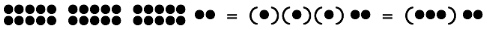# Depth-value Notation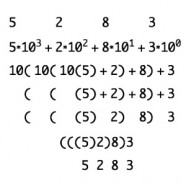Due to the huge inconvenience of having to count large numbers of units, unit arithmetic gave way to named groups, which then evolved into common base systems and to place-value notation.  This last innovation, place-values, occurred before the advent of computers, in an era when sequential math was dominant. Depth-value notation provides the advantages of our common place-value notation while incorporating three additional benefits. Depth value maintains the Additive Principle, it greatly simplifies the way numbers are combined (the addition and multiplication algorithms), and it facilitates parallel processing of each grouping of numbers.  The Image shows the polynomial, place-value form of the number 5283 and the factored, depth-value form. The fundamental innovation of depth-value notation is to use a representation for numbers that has both an inside and an outside.  Parentheses, ( ), and other delimiting tokens provide this inside/outside structure, as do planar enclosures such as circles and rectangles.  The boundary created by a container such as the parenthesis serves to keep track of magnitude independently of addition and multiplication operations. Crossing the boundary from inside to outside can be read as multiplying the interior contents by one order of magnitude. A unit or numeral on the outside of a boundary is sharing the same space as the bounded number and thus is being added due to the Additive Principle.

## Place-value Notation

The positional notation of our number system is considered to be one of humankind’s greatest achievements.  It allows us to express large numbers using only ten digits, together with a convention that moving a digit one place to the left of its current position multiplies its value by 10.  The key to place-value notation is that the same ten digits can be reused in different places to convey different magnitudes.  This works particularly well when we adopt a uniform base, when all positions multiply by the same number. The digit zero is a necessary place holder, in case there are no units in a particular multiple of ten.  By including a decimal point to serve as an one-dimensional origin, we can stack digits to the right to express values divided by ten. Thus positional notation uses two zero concepts, the place-holder zero, 0, and the unit origin decimal point, . .

Place-value is the product of pragmatic compromise.  Arabic-numbers exchange a great gain in readability for a moderate loss in computability.  They solve the problem of being able to identify large ensembles by the cardinal value, or size, but they are more difficult to combine, to add and to multiply. Numbers no longer add by mere spatial presence, instead they require both memorization of number facts (such as 7+5=12) and tracking the place-value of each digit.  Digits must be maintained in a strict sequential position, forcing sequential thinking about how numbers work. Calculation includes techniques for interfacing adjacent sequential positions, commonly called “carrying” and “borrowing”.  It takes several years of schooling for young students to master these tools.

Another way to characterize place-value notation is to say that numerals are polynomials.  Abstractly a four-digit number ABCD is shorthand for the polynomial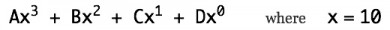The number 10 is called the base of the system.  A uniform base makes positional notation easier to compute with, and thus increases the efficiency of bookkeeping, banking and measurement. As a specific example, the positional number 5083 is shorthand for a specific polynomial with x=10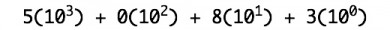For all of its magnificent advantages, positional notation imposes several significant costs, the greatest of which is that it no longer supports the intuitive Additive Principle.  Instead of simply putting together, we need to use complicated algorithms to be able to add and to multiply.  These algorithms are sufficiently difficult that it takes most humans half a decade of practice, throughout elementary school, to master them.  Place-value algorithms thoroughly mix arithmetic operations with the maintenance of the place-value notation. Students and teachers are thus led to believe that addition is defined by the three mechanisms that maintain place-value: digit combination facts, alignment of place-values, and carrying. Digit combination facts are memorized as operations that permit two digit symbols to be replaced by one digit and a possible carry operation. The addition facts are simply symbol reference tables associated with notational abbreviations, and not with the objects or concepts of addition. Carrying changes the value of a positional neighbor when digit addition facts generate a numerical result that is greater than any single digit. Alignment of place-value is the collection of like terms by distribution, part of the process of polynomial simplification. In the shorthand of place-value notation, like terms refers to a property shared by all digits that belong to a particular order of magnitude (i.e. the same column, the same polynomial degree of the base).

Symbolic addition is purely syntactic. Substituting numerals provides notational convenience; aligning columns maintains the place-value decomposition of number; and carrying base overflows is a pragmatic trade-off between how many number-facts the base system requires and the frequency of having to adjust for overflow. The pedagogical shift from early childhood manipulatives to symbolic computation is a shift from semantics to syntax, from construction of meaning to management of a particular representation. Our current approach to teaching and learning arithmetic cannot make sense because symbolic algorithms maintain meaning (i.e. the value of an expression) but do not embody meaning. When we compute with symbols, we leave meaning and worldly experience at the door.

## Depth-value Notation

Depth-value notation achieves the same advantages as place-value notation, while maintaining the Additive Principle, while reducing the number of needed addition facts, while maintaining parallelism so that each depth can be summed at the same time, and while allowing as many positive and negative numbers as desired to be added  and subtracted at the same time. The Iconic Calculator illustrates these advantages.

One way to express depth-value notation is to say that numerals are factored polynomials.  The four digit number ABCD is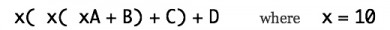The example number 5083 would look like this: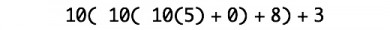As is the case with place-value, we do not have to write the base-10 explicitly.  Rather than saying “multiply by ten every time you move one position to the left”, we can say “multiply by ten every time you cross a boundary outwards”.  Note that the parentheses have taken on an entirely different meaning. They actively multiply their contents rather than passively group their contents. Below, instead of writing the factor 10, we can adopt the convention to read parentheses as a notation for magnitude.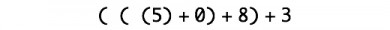Nor do we need to write the plus sign.  Numbers sharing the same space are added together, that’s what adding means.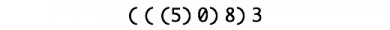We also no longer need the zero place-holder because the depth boundaries themselves keep track of nothing.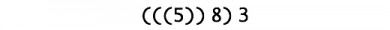Finally, to get back to our conventional notation, just erase the boundaries, adding zero where necessary.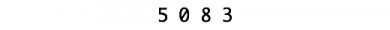Why go to the trouble of using depth boundaries rather than left/right positions?  Because depth-value numerals are iconic.  They are much easier to learn and to use, and they can be physically manipulated rather than memorized.  Depth-value transfers the computation of place-value addition and multiplication algorithms to two simple standardization rules. And arithmetic begins its journey back to comfort and comprehension.

The following two rules, Grouping and Merging, are all that is needed to maintain depth-value notation.

## Standardization

When unit-ensembles are added, they are placed together in the same space. Depth-value notation simplifies large collections of units through grouping, similar to drawing a bar across four strokes to achieve a group of five in tally notation and similar to replacing five strokes by the V of Roman numerals. However, in tallies and in Roman numerals, there is no convenient mechanism to combine groups.  Depth-value includes a simple mechanism to combine numbers of the same magnitude together, analogous to keeping track of the place-value columns for conventional numerals, but permitting all steps to be taken in parallel.

### Grouping

A group of units outside a container or boundary can be represented by a single unit inside the container.  The container itself multiplies its contents. How many units are grouped together is determined by the base of the number system.  For the common base-10 the Grouping Rule is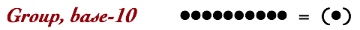The boundary multiplies its contents by 10.  Enclosure by a boundary is equivalent to shifting the decimal point one column to the right.  The grouping rule for base-2 is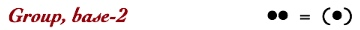### Merging

Boundaries are combined by merging, that is, by erasing edges that are in contact.  For all bases the Merging Rule is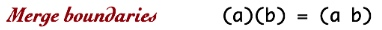Merging is equivalent to lining up columns for conventional addition, but with significant advantages. Boundaries automatically keep track of the orders of magnitude, there is no possibility of misaligning numbers. Merging occurs by deletion of edges; erasure is a very easy mechanism to understand. Boundaries are physical and manipulable, there is little to memorize and to remember. Learning can be visual and tactile. Merging can occur in parallel.  And importantly, boundary merging shows the process of grouping, it can be seen, felt, and trusted.

Here is a base-10 example of both rules working to reduce 32 individual units to the depth-value notation for 32.